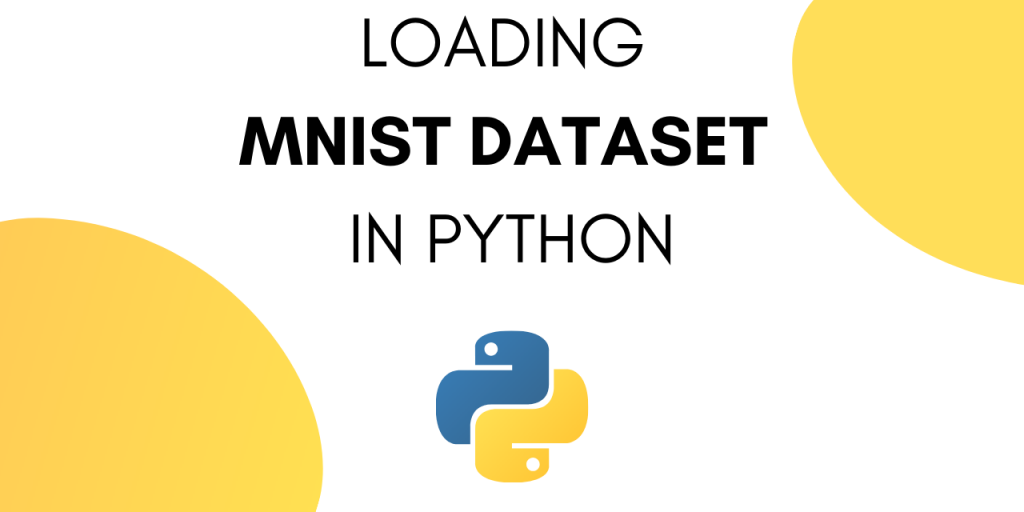# How to Load and Plot the MNIST dataset in Python?This tutorial covers the step to load the MNIST dataset in Python. The MNIST dataset is a large database of handwritten digits. It commonly used for training various image processing systems.

MNIST is short for Modified National Institute of Standards and Technology database.

This dataset is used for training models to recognize handwritten digits. This has an application in scanning for handwritten pin-codes on letters.

MNIST contains a collection of 70,000, 28 x 28 images of handwritten digits from 0 to 9.

## Why is MNIST dataset so popular?

MNIST is popular for a multitude of reasons, these are :

• MNSIT dataset is publicly available.
• The data requires little to no processing before using.
• It is a voluminous dataset.

Additionally, this dataset is commonly used in courses on image processing and machine learning.

In this tutorial, we will be learning about the MNIST dataset. We will also look at how to load the MNIST dataset in python.

Let’s start by loading the dataset into our python notebook. The easiest way to load the data is through Keras.

```from keras.datasets import mnist
```

MNIST dataset consists of training data and testing data. Each image is stored in 28X28 and the corresponding output is the digit in the image.

We can verify this by looking at the shape of training and testing data.

To load the data into variables use:

```(train_X, train_y), (test_X, test_y) = mnist.load_data()
```

To print the shape of the training and testing vectors use :

```print('X_train: ' + str(train_X.shape))
print('Y_train: ' + str(train_y.shape))
print('X_test:  '  + str(test_X.shape))
print('Y_test:  '  + str(test_y.shape))
```

We get the following output :

```X_train: (60000, 28, 28)
Y_train: (60000,)
X_test:  (10000, 28, 28)
Y_test:  (10000,)
```

From this we can conclude the following about MNIST dataset :

• The training set contains 60k images and the testing set contains 10k images.
• The training input vector is of the dimension [60000 X 28 X 28].
• The training output vector is of the dimension [60000 X 1].
• Each individual input vector is of the dimension [28 X 28].
• Each individual output vector is of the dimension .

### 2. Plotting the MNIST Dataset

Let’s try displaying the images in the MNIST dataset. Start by importing Matplotlib.

```from matplotlib import pyplot
```

To plot the data use the following piece of code :

```from matplotlib import pyplot
for i in range(9):
pyplot.subplot(330 + 1 + i)
pyplot.imshow(train_X[i], cmap=pyplot.get_cmap('gray'))
pyplot.show()
```

The output comes out as :

## Complete Code to Load and Plot MNIST Dataset in Python

The complete code for this tutorial is given below:

```from keras.datasets import mnist
from matplotlib import pyplot

(train_X, train_y), (test_X, test_y) = mnist.load_data()

#shape of dataset
print('X_train: ' + str(train_X.shape))
print('Y_train: ' + str(train_y.shape))
print('X_test:  '  + str(test_X.shape))
print('Y_test:  '  + str(test_y.shape))

#plotting
from matplotlib import pyplot
for i in range(9):
pyplot.subplot(330 + 1 + i)
pyplot.imshow(train_X[i], cmap=pyplot.get_cmap('gray'))
pyplot.show()

```

## What’s next?

Now that you have imported the MNIST dataset, you can use it for image classification.

When it comes to the task of image classification, nothing can beat Convolutional Neural Networks (CNN). CNN contains Convolutional Layers, Pooling Layers, and Flattening Layers.

Let’s see what each of these layers do.

### 1. Convolution Layer

Convolution layer filters the image with a smaller pixel filter. This decreases the size of the image without losing the relationship between pixels.

### 2. Pooling Layer

The main job of the pooling layer is to reduce the spatial size of the image after convolution.

A pooling layer reduces the amount of parameters by selecting the maximum, average, or sum values inside the pixels.

Max pooling is the most commonly used pooling technique.

### 3. Flattening Layer

A flattening layer represents the multi-dimensional pixel vector as a one-dimensional pixel vector.

## Conclusion

This tutorial was about loading MNIST Dataset into python. We explored the MNIST Dataset and discussed briefly about CNN networks that can be used for image classification on MNIST Dataset.

If you’d like to learn further about processing images in Python, read through this tutorial on how to read images in Python using OpenCV.